## Chapter 3 Pair of Linear Equations in Two Variables R.D. Sharma Solutions for Class 10th Math Exercise 3.8

Exercise 3.8

1. The numerator of a fraction is 4 less than the denominator. If the numerator is decreased by 2 and denominator is increased by 1, then the denominator is eight times the numerator. Find the fraction.

Solution

Let the numerator and denominator of the fraction be x and y respectively. Then the fraction is x/y
The numerator of the fraction is 4 less the denominator. Thus, we have
x = y – 4
⇒ x – y = -4
If the numerator is decreased by 2 and denominator is increased by 1, then the denominator is 8 times the numerator. Thus, we have
y + 1 = 8(x-2)
⇒ y + 1 = 8x – 16
⇒ 8x – y = 1 + 16
⇒ 8x – y = 17
So, we have two equations
x – y = -4
8x – y = 17
Here, x and y are unknowns. We have to solve the above equations for x and y.
Subtracting the second equation from the first equation, we get
(x-y) – (8x – y) = -4 -17
⇒ x – y - 8x + y = -21
⇒ - 7x = -21
⇒ 7x = 21
⇒ x = 21/7
⇒ x = 3
Substituting the value of x in the first equation , we have
3 – y = -4
⇒ y = 3 + 4
⇒ y =7
Hence, the fraction is 3/7.

2. A fraction becomes 9/11 if 2 is added to both numerator and the denominator. If 3 is added to both the numerator and the denominator it becomes 5/6. Find the fraction.

Solution

Let the numerator and denominator of the fraction be x and y respectively. Then the fraction is x/y
If 2 is added to both numerator and the denominator, the fraction becomes 9/11 . Thus, we have
x+2/y+2 = 9/11
⇒ 11(x+2) = 9 (y+2)
⇒ 11x + 22 = 9(y+2)
⇒ 11x + 22 = 9y + 18
⇒ 11x – 9y = 18 – 22
⇒ 11x – 9y + 4 = 0
If 3 is added to both numerator and the denominator, the fraction becomes 5/6 . Thus , we have
x + 3/y + 3 = 5/6
⇒ 6(x+3) = 5 (y+3)
⇒ 6x + 18 = 5y + 15
⇒ 6x – 5y = 15 - 18
⇒ 6x – 5y + 3 = 0
So, we have two equations
11x -9y + 4 =0
6x -5y + 3 = 0
Here, x and y are unknowns. We have to solve the above equations for x and y.
By using cross-multiplication, we have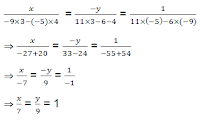⇒ x = 7, y = 9
Hence, the fraction is 7/9

3. A fraction becomes 1/3 if 1 is subtracted from both its numerator and denominator. It 1 is added to both the numerator and denominator, it becomes 1/2. Find the fraction.

Solution

Let the numerator and denominator of the fraction be x and y respectively. Then the fraction is x/y .
If 1 is substracted from both numerator and the denominator, the fraction becomes 1/3.
x-1/y -1 = 1/3
⇒ 3(x-1) = y-1
⇒ 3x – 3 = y -1
⇒ 3x – y – 2 = 0
If 1 is added to both numerator and the denominator, the fraction becomes 1/2 .
x+1/y +1 = 1/2
⇒ 2(x+1) = y+1
⇒ 2x + 2 = y + 1
⇒ 2x – y + 1 = 0
So, we have two equations
3x – y – 2 = 0
2x – y + 1 = 0
Here, x and y are unknowns. We have to solve the above equations for x and y.
By using cross – multiplication, we have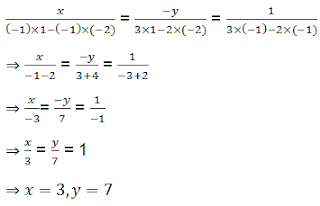Hence, the fraction is 3/7 .

4. If we add 1 to the numerator and subtract 1 from the denominator, a fraction becomes 1. It also becomes 1/2 if we only add 1 to the denominator. What is the fraction ?

Solution

Let the numerator and denominator of the fraction be x and y respectively. Then the fraction is x/y .
If 1 is added to the numerator and 1 is subtracted from the denominator, the fraction becomes 1. Thus,  we have
x+1/y -1 = 1
⇒x + 1 = y – 1
⇒ x + 1 – y + 1 = 0
⇒ x – y + 2 = 0
If 1 is added to the denominator , the fraction becomes 1/2 . Thus, we have
x/y+1 = ½
⇒ 2x = y + 1
⇒ 2x – y – 1 = 0
So, we have two equations
x – y + 2 = 0
2x – y – 1 = 0
Here, x and y are unknowns. We have to solve the above equations for x and y.
By using cross-multiplication, we have5. If the numerator of a fraction is multiplied by 2 and the denominator is reduced by 5 the fraction becomes 6/5. And, if the denominator is doubled and the numerator is increased by 8, the fraction becomes 2/5. find the fraction.

Solution

Let the numerator and denominator of the fraction be x and y respectively. Then the fraction is x/y
If the numerator is multiplied by 2 and the denominator is reduced by 5, the fraction becomes 6/5 . Thus, we have
2x/y–5 = 6 / 5
⇒ 10x = 6 (y-5)
⇒ 10x = 6y – 30
⇒ 10x – 6y + 30 = 0
⇒ 2 (5x – 3y + 15) = 0
⇒ 5x – 3y + 15 = 0
If the denominator is doubled and the numerator is increased by 8, the fraction becomes 2/5 . Thus, we have
x+8/2y = 2/5
⇒ 5(x+8) = 4y
⇒ 5x + 40 = 4y
⇒ 5x – 4y + 40 = 0
So, we have two equations
5x – 3y + 15 = 0
5x – 4y + 40 = 0
Here, x and y are unknowns. We have to solve the above equations for x and y.
By using cross-multiplication, we have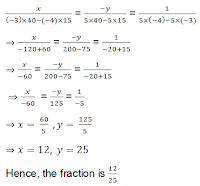6. When 3 is added to the denominator and 2 is subtracted from the numerator a fraction becomes 1/4. And when 6 is added to numerator and the denominator is multiplied by 3, it becomes 2/3. Find the fraction.

Solution

Let the numerator and denominator of the fraction be x and y respectively. Then the fraction is x/y .
If 3 is added to the denominator and 2 is subtracted from the numerator , the fraction becomes 1/4.
Thus , we have
x-2/y+3 = 1/4
⇒ 4(x-2) = y+3
⇒ 4x – 8 = y + 3
4x – y – 11 = 0
If 6 is added to the numerator and the denominator is multiplied by 3, the fraction becomes 2/3 . Thus, we have
x+6/3y = 2/3
⇒ 3(x+6) = 6y
⇒ 3x + 18 = 6y
⇒ 3x – 6y + 18 = 0
⇒ 3(x - 2y + 6) = 0
⇒ x – 2y + 6 = 0
So, we have two equations
4x – y – 11 = 0
x – 2y + 6 = 0
Here, x and y are unknowns. We have to solve the above equations for x and y.
By using cross-multiplication, we have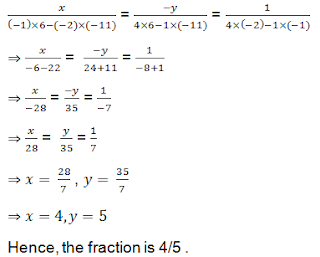7. The sum of a numerator and denominator of a fraction is 18. If the denominator is increased by 2, the fraction reduces to 1/3. Find the fraction.

Solution

Let the numerator and denominator of the fraction be x and y respectively. Then the fraction is x/y
The sum of the numerator and the denominator of the fraction is 18. Thus, we have
x + y = 18
⇒ x + y – 18 = 0
If the denominator is increased by 2, the fraction reduces by 2, the fraction reduces to 1/3 . Thus, we have
x/y+2 = 1/3
⇒ 3x = y + 2
⇒ 3x – y – 2 = 0
So, we have two equations
x + y – 18 = 0
3x – y – 2 = 0
Here, x and y are unknowns. We have to solve the above equations for x and y.

By using cross-multiplication, we have8. If 2 is added to the numerator of a fraction, it reduces to 1/2 and if 1 is subtracted from the denominator, it reduces to 1/3. Find the fraction.

Solution

Let the numerator and denominator of the fraction be x and y respectively. Then the fraction is x/y
If 2 is added to the numerator of the fraction, it reduces to 1/2 . Thus, we have
x+2/y = 1/2
⇒ 2(x+2) = y
⇒ 2x + 4 = y
⇒ 2x – y + 4 = 0
If 1 is subtracted from the denominator, the fraction reduces to 1/3 . Thus, we have
x/y – 1 = 1/3
⇒ 3x = y – 1
⇒ 3x – y + 1 = 0
So, we have two equations
2x –y + 4 = 0
3x – y + 1 = 0
Here x and y are unknowns . We have to solve the above equations for x and y .
By using cross – multiplicatiion , we have9. The sum of the numerator and denominator of a fraction is 4 more than twice the numerator. If the numerator and denominator are increased by 3, they are in the ratio 2 : 3. Determine the fraction.

Solution

Let the numerator and denominator of the fraction be x and y respectively. Then the fraction is x/y
The sum of the numerator and denominator of the fraction is 4 more than twice the numerator. Thus, we have
x+y = 2x + 4
⇒ 2x + 4 – x – y = 0
⇒ x – y + 4 = 0
If the numerator and denominator are increased by 3, they are in the ratio 2:3. Thus, we have
x + 3 : y + 3 = 2 : 3
⇒ x + 3 / y + 3 = 2/3
⇒ 3(x+3) = 2(y+3)
⇒ 3x + 9 = 2y + 6
⇒ 3x – 2y + 3 = 0
So, we have two equations
x – y + 4 = 0
3x – 2y + 3 = 0
Here x and y are unknowns. We have to solve the above equations for x and y. By using cross-multiplication, we have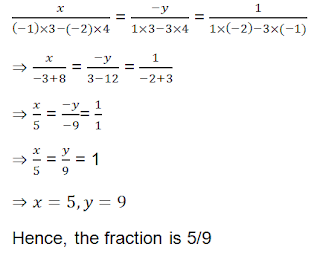10. The sum of the numerator and denominator of a fraction is 3 less than twice the denominator. If the numerator and denominator are decreased by 1, the numerator becomes half the denominator. Determine the fraction.

Solution

Let the numerator and denominator of the fraction be x and y respectively. Then the fraction is x/y
The sum of the numerator and denominator of the fraction is 3 less than twice the denominator.
Thus, we have
x+y = 2y – 3
⇒ x+y-2y+3 = 0
⇒ x–y+3= 0
If the numerator and denominator are decreased by 1, the numerator becomes half the denominator. Thus, we have
x-1 = 1/2 (y-1)
⇒ x-1/y-1 =1/2
⇒ 2(x-1) = y-1
⇒ 2x-2=y-1
⇒ 2x-y-1=0
So, we have two equations
x-y+3=0
2x-y-5=0
Here x and y are unknowns . We have to solve the above equations for x and y.
By using cross – multiplication, we have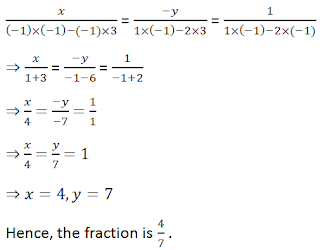11. The sum of the numerator and denominator of a fraction is 12. If the denominator is increased by 3, the fraction becomes 1/2. Find the fraction.

Solution

Let the numerator and denominator of the fraction be x and y respectively. Then the fraction is x/y The sum of the numerator and denominator of the fraction is 12. Thus, we have
x+y=12
⇒ x+y-12=0
If the denominator is increased by 3, the fraction becomes 1/2. Thus, we have
x/y+3 = 1/2
⇒ 2x = y + 3 = 0
⇒ 2x – y – 3 = 0
So, we have two equations
x + y – 12 = 0
2x – y – 3 = 0
Here x and y unknowns . We have to solve the above equations for x and y. By using cross – multiplication, we have